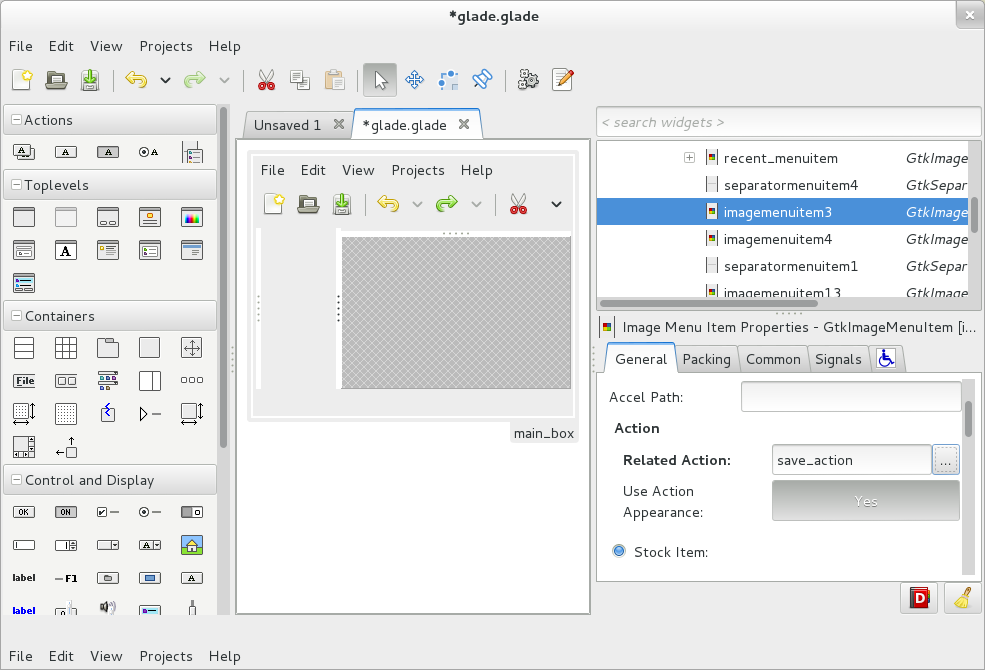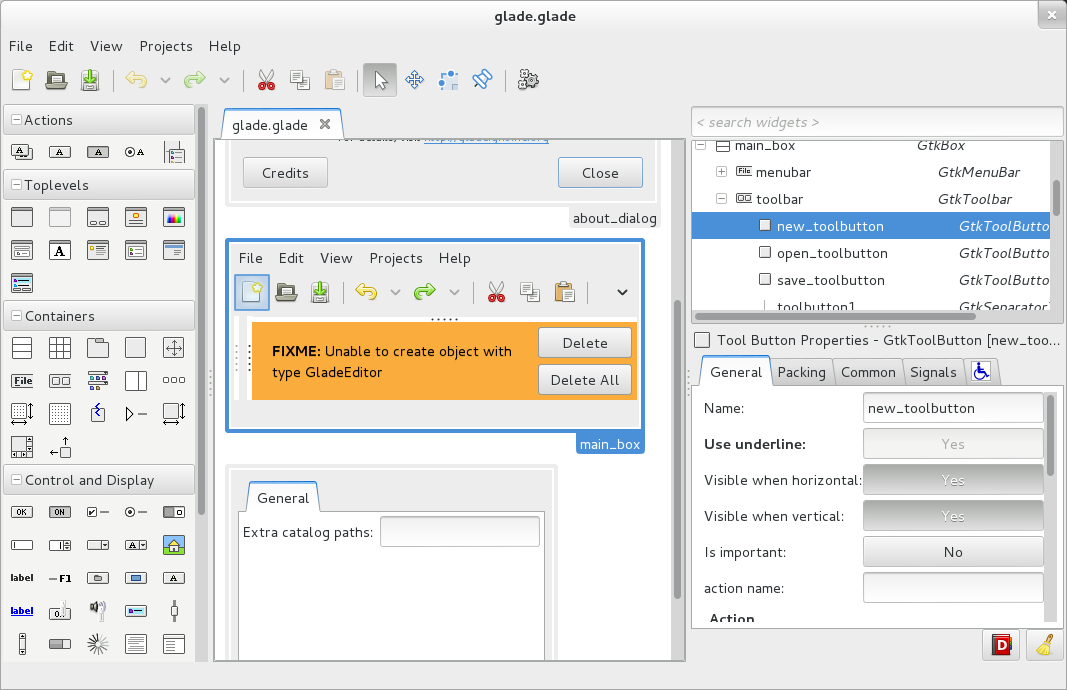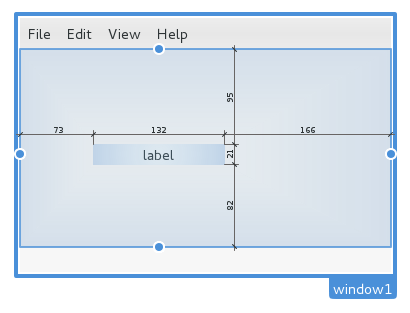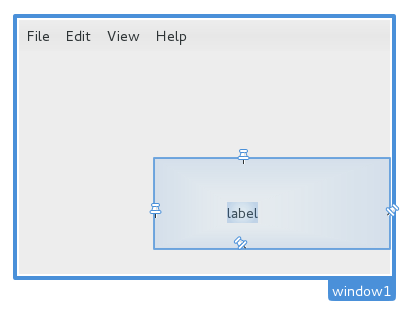## Cross compiling Glade for win32

It was a long time since I did not give cross compiling a try and it turns out to be easier than expected if you use precompiled binaries from windows:mingw project on the OpenSUSE Build Service. (OBS)

First of all we need to install the cross compiler, in Debian and Debian based distros do

`\$ sudo apt-get install mingw-w64`

`wget https://github.com/mkbosmans/download-mingw-rpm/raw/master/download-mingw-rpm.py`

Now we can download the dependencies (hicolor-icon-theme is only needed at runtime)

```python3 download-mingw-rpm.py --deps gtk3-devel libxml2-devel \
hicolor-icon-theme```

This will unpack everything under usr/i686-w64-mingw32/sys-root/mingw

Next we need to setup some variables for the cross compilation

```export CC=i686-w64-mingw32-gcc
export PKG_CONFIG_LIBDIR=\$MINGW_ROOT/lib/pkgconfig
export PKG_CONFIG_PATH=\$MINGW_ROOT/share/pkgconfig
export PKG_CONFIG="pkg-config --define-variable=prefix=\$MINGW_ROOT"```

CC: the cross compiler
MINGW_ROOT: is an absolute path we are going to use as a prefix for our build
PKG_CONFIG_LIBDIR: this way we tell pkg-config to use mingw libs
PKG_CONFIG_PATH: just to make sure we are not pointing some where else
PKG_CONFIG: ask pkg-config to replace .pc files prefix variable with the real path

And this is pretty much it, now we can proceed to run autogen.sh with –host and –target flags and glade should compile

```git clone git://git.gnome.org/glade
./autogen.sh --prefix=\$MINGW_ROOT --host i686-w64-mingw32 \
--target i686-w64-mingw32 --enable-shared=yes --enable-static=no
make
make install```

Before you can run it you need to update glib schemas bin file

`glib-compile-schemas \$MINGW_ROOT/share/glib-2.0/schemas`

BTW there is a scrip file that automates this process and creates a windows installer using nsis
in glade sources under build/mingw-w64 directory.

## SVG && cairo_path_t fun!

For all of those of you who usually program with cairo: How do you include a somewhat complex path in your code?

Let’s take GIMP as an example…

Wilber figure shows ups in a few places and the cairo code to draw it is in one place

http://git.gnome.org/browse/gimp/tree/app/widgets/gimpcairo-wilber.c

The important function here is `gimp_cairo_wilber`() which uses a path created from a SVG path description string. This allows them to grab that string and paste it inside a SVG file and edit it with Inkscape for example. Which could be annoying if you have to do it frequently or if it has to be done by an artist that does not feel comfortable messing around with source code.

This is the SVG path for wilber (WordPress does not allow me to include a svg image)

``` <svg width="225" height="165">   <g transform="translate(-287,-438)">     <path id="path2987" d="m 509.72445,438.68864 c -8.24739,31.09081 -44.77407,52.85702 -77.8653,59.0601 6.66245,5.26814 11.01867,13.47826 11.01867,22.62501 1e-5,15.87371 -12.92175,28.64855 -28.79547,28.64855 -15.87372,0 -28.79547,-12.77484 -28.79547,-28.64855 0,-8.84972 3.98978,-16.76089 10.2841,-22.03735 -36.20146,-2.43256 -51.86122,-34.37828 -51.86123,-34.37826 l -1.02841,45.69077 c 0,4.7013 -0.59743,10.31207 -2.49756,18.65829 -0.33714,-0.50356 -0.66979,-0.97205 -1.02841,-1.46916 -8.90026,-12.33694 -21.90268,-19.02373 -32.7622,-18.07063 -3.61983,0.3177 -6.923,1.56607 -9.84335,3.67289 -11.68135,8.42727 -11.57317,28.50691 0.29384,44.9562 10.11908,14.02637 25.47866,20.85962 37.02274,17.33604 58.07995,40.4437 198.30291,67.68661 175.85805,-136.0439 z M 363.24953,501.1278 c 10.58249,-2e-5 19.24596,8.66347 19.24596,19.24595 0,10.58249 -8.66348,19.09904 -19.24596,19.09904 -10.58247,0 -19.09903,-8.51655 -19.09903,-19.09904 -1e-5,-10.58246 8.51656,-19.24595 19.09903,-19.24595 z m -57.44402,14.9854 c 5.87915,-2e-5 10.57793,5.72665 10.57793,12.78166 10e-6,7.05496 -4.69877,12.78166 -10.57793,12.78166 -5.87915,0 -10.72484,-5.72665 -10.72484,-12.78166 -2e-5,-7.05501 4.84569,-12.78166 10.72484,-12.78166 z M 440.821,552.54828 c 0,0 7.9294,1.4756 13.0755,6.90504 3.52231,3.71619 3.85558,9.70174 3.08522,17.92371 -0.77029,-3.49373 -2.08601,-5.61044 -3.08522,-8.08037 -10.88262,13.17996 -40.46669,13.79263 -77.8653,0.58767 40.60128,8.1206 61.35686,0.67581 73.45783,-8.66803 -3.1952,-4.12713 -8.66803,-8.66802 -8.66803,-8.66802 z m -6.17377,-27.95144 c 0,7.6429 -6.20294,13.84584 -13.84584,13.84584 -7.6429,0 -13.84584,-6.20294 -13.84584,-13.84584 0,-7.6429 6.20294,-13.84584 13.84584,-13.84584 7.6429,0 13.84584,6.20294 13.84584,13.84584 z m -56.6468,-1.59753 c 0,4.70333 -3.81719,8.52053 -8.52052,8.52053 -4.70333,0 -8.52052,-3.8172 -8.52052,-8.52053 0,-4.70332 3.81719,-8.52052 8.52052,-8.52052 4.70333,0 8.52052,3.8172 8.52052,8.52052 z"/>   </g> </svg```

So after doing the same thing a few times for Glade

I decided to automate the process by creating a simple application that takes a SVG file and outputs C code for a cairo_path_t struct.

```#ifndef __WILBER_H__ #define __WILBER_H__   #define WILBER_WIDTH 225.000000 #define WILBER_HEIGHT 165.000000 extern cairo_path_t wilber_path;   #endif /* __WILBER_H__ */```
```#include <cairo.h>

static cairo_path_data_t wilber_data[] = {
{.point.x = 509.724450, .point.y = 438.688640},
{.point.x = 501.477060, .point.y = 469.779450},
{.point.x = 464.950380, .point.y = 491.545660},
{.point.x = 431.859150, .point.y = 497.748740},
{.point.x = 438.521600, .point.y = 503.016880},
{.point.x = 442.877820, .point.y = 511.227000},
{.point.x = 442.877820, .point.y = 520.373750},
{.point.x = 442.877830, .point.y = 536.247460},
{.point.x = 429.956070, .point.y = 549.022300},
{.point.x = 414.082350, .point.y = 549.022300},
{.point.x = 398.208630, .point.y = 549.022300},
{.point.x = 385.286880, .point.y = 536.247460},
{.point.x = 385.286880, .point.y = 520.373750},
{.point.x = 385.286880, .point.y = 511.524030},
{.point.x = 389.276660, .point.y = 503.612860},
{.point.x = 395.570980, .point.y = 498.336400},
{.point.x = 359.369520, .point.y = 495.903840},
{.point.x = 343.709760, .point.y = 463.958120},
{.point.x = 343.709750, .point.y = 463.958140},
{.point.x = 342.681340, .point.y = 509.648910},
{.point.x = 342.681340, .point.y = 514.350210},
{.point.x = 342.083910, .point.y = 519.960980},
{.point.x = 340.183780, .point.y = 528.307200},
{.point.x = 339.846640, .point.y = 527.803640},
{.point.x = 339.513990, .point.y = 527.335150},
{.point.x = 339.155370, .point.y = 526.838040},
{.point.x = 330.255110, .point.y = 514.501100},
{.point.x = 317.252690, .point.y = 507.814310},
{.point.x = 306.393170, .point.y = 508.767410},
{.point.x = 302.773340, .point.y = 509.085110},
{.point.x = 299.470170, .point.y = 510.333480},
{.point.x = 296.549820, .point.y = 512.440300},
{.point.x = 284.868470, .point.y = 520.867570},
{.point.x = 284.976650, .point.y = 540.947210},
{.point.x = 296.843660, .point.y = 557.396500},
{.point.x = 306.962740, .point.y = 571.422870},
{.point.x = 322.322320, .point.y = 578.256120},
{.point.x = 333.866400, .point.y = 574.732540},
{.point.x = 391.946350, .point.y = 615.176240},
{.point.x = 532.169310, .point.y = 642.419150},
{.point.x = 509.724450, .point.y = 438.688640},
{.point.x = 363.249530, .point.y = 501.127800},
{.point.x = 373.832020, .point.y = 501.127780},
{.point.x = 382.495490, .point.y = 509.791270},
{.point.x = 382.495490, .point.y = 520.373750},
{.point.x = 382.495490, .point.y = 530.956240},
{.point.x = 373.832010, .point.y = 539.472790},
{.point.x = 363.249530, .point.y = 539.472790},
{.point.x = 352.667060, .point.y = 539.472790},
{.point.x = 344.150500, .point.y = 530.956240},
{.point.x = 344.150500, .point.y = 520.373750},
{.point.x = 344.150490, .point.y = 509.791290},
{.point.x = 352.667060, .point.y = 501.127800},
{.point.x = 363.249530, .point.y = 501.127800},
{.point.x = 305.805510, .point.y = 516.113200},
{.point.x = 311.684660, .point.y = 516.113180},
{.point.x = 316.383440, .point.y = 521.839850},
{.point.x = 316.383440, .point.y = 528.894860},
{.point.x = 316.383450, .point.y = 535.949820},
{.point.x = 311.684670, .point.y = 541.676520},
{.point.x = 305.805510, .point.y = 541.676520},
{.point.x = 299.926360, .point.y = 541.676520},
{.point.x = 295.080670, .point.y = 535.949870},
{.point.x = 295.080670, .point.y = 528.894860},
{.point.x = 295.080650, .point.y = 521.839850},
{.point.x = 299.926360, .point.y = 516.113200},
{.point.x = 305.805510, .point.y = 516.113200},
{.point.x = 440.821000, .point.y = 552.548280},
{.point.x = 440.821000, .point.y = 552.548280},
{.point.x = 448.750400, .point.y = 554.023880},
{.point.x = 453.896500, .point.y = 559.453320},
{.point.x = 457.418810, .point.y = 563.169510},
{.point.x = 457.752080, .point.y = 569.155060},
{.point.x = 456.981720, .point.y = 577.377030},
{.point.x = 456.211430, .point.y = 573.883300},
{.point.x = 454.895710, .point.y = 571.766590},
{.point.x = 453.896500, .point.y = 569.296660},
{.point.x = 443.013880, .point.y = 582.476620},
{.point.x = 413.429810, .point.y = 583.089290},
{.point.x = 376.031200, .point.y = 569.884330},
{.point.x = 416.632480, .point.y = 578.004930},
{.point.x = 437.388060, .point.y = 570.560140},
{.point.x = 449.489030, .point.y = 561.216300},
{.point.x = 446.293830, .point.y = 557.089170},
{.point.x = 440.821000, .point.y = 552.548280},
{.point.x = 440.821000, .point.y = 552.548280},
{.point.x = 434.647230, .point.y = 524.596840},
{.point.x = 434.647230, .point.y = 532.239740},
{.point.x = 428.444290, .point.y = 538.442680},
{.point.x = 420.801390, .point.y = 538.442680},
{.point.x = 413.158490, .point.y = 538.442680},
{.point.x = 406.955550, .point.y = 532.239740},
{.point.x = 406.955550, .point.y = 524.596840},
{.point.x = 406.955550, .point.y = 516.953940},
{.point.x = 413.158490, .point.y = 510.751000},
{.point.x = 420.801390, .point.y = 510.751000},
{.point.x = 428.444290, .point.y = 510.751000},
{.point.x = 434.647230, .point.y = 516.953940},
{.point.x = 434.647230, .point.y = 524.596840},
{.point.x = 378.000430, .point.y = 522.999310},
{.point.x = 378.000430, .point.y = 527.702640},
{.point.x = 374.183240, .point.y = 531.519840},
{.point.x = 369.479910, .point.y = 531.519840},
{.point.x = 364.776580, .point.y = 531.519840},
{.point.x = 360.959390, .point.y = 527.702640},
{.point.x = 360.959390, .point.y = 522.999310},
{.point.x = 360.959390, .point.y = 518.295990},
{.point.x = 364.776580, .point.y = 514.478790},
{.point.x = 369.479910, .point.y = 514.478790},
{.point.x = 374.183240, .point.y = 514.478790},
{.point.x = 378.000430, .point.y = 518.295990},
{.point.x = 378.000430, .point.y = 522.999310},
};

cairo_path_t wilber_path = {0, wilber_data, 160};```

Which can be easily integrated in the Makefile system with a few rules like

```# Rules to generate cairo paths %.h: %.svg cairo_svg2path \$< --target=\$@ %.c: %.svg cairo_svg2path \$< --target=\$@```

Then all you have to do is include the corresponding header and use
`cairo_append_path (cr, &wilber_path);`
to append the path to a cairo context.

You can find cairo_svg2path source code in cairo bugzilla page
https://bugs.freedesktop.org/show_bug.cgi?id=50363
Enjoy!

Last week, inspired by Tristan’s GtkComposite branch idea of embedding builder xml into widget classes together with the old eagerness  of implementing Glade UI with Glade itself I started hacking Glade in glade!

The first step was to recreate GladeWindow widget hierarchy in Glade, that was quick and easy. Then I started replacing the hard coded widgets with it but since I wanted to use the very same executable I was hacking on to edit its own UI definition file I had to make sure I got the palette, design view, inspector and property editor properly working before removing the old code.Later on I proceeded to remove the old menu that was implemented using GtkUIManager… big mistake! How was I supposed to edit the file if I was not able to save the file, so I revert it and moved it to the end of the window and keep it until the new menu had a working save menu item!

After this, Glade was functional and only had one regression. For some reason GtkAccelLabel does not show up the accelerator when used with a related action.  But that seems to be a Gtk+ bug (see bugzilla report)The only problem left to solve was the dependency GladeWindow had on the UI file. I really did not like the idea of a class depending on a file so I decided it was a good time to give GResource a try! GResource allows you to easily embed resources into your code. All you have to do is define all your resources in GResource xml format, in this case only one file glade.glade.

```<?xml version="1.0" encoding="UTF-8"?> <gresources> <gresource prefix="/org/gnome/glade"> <file compressed="true">glade.glade</file> </gresource> </gresources>```

And compile it into source using `glib-compile-resourses` tool, which can be done automatically with a couple of Makefile rules.

```# Rules to compile resources %.h: %.gresource.xml   \$(GLIB_COMPILE_RESOURCES) --generate \$< --target=\$@ %.c: %.gresource.xml   \$(GLIB_COMPILE_RESOURCES) --generate \$< --target=\$@   # Dependencies rule, and do not forget to do a make after # editing the UI with glade! glade-resources.c glade-resources.h: \   glade-resources.gresource.xml \   \$(shell \$(GLIB_COMPILE_RESOURCES) --generate-dependencies \    glade-resources.gresource.xml)```

Once you have the build system setup it is as simply as using the resource uri instead of a file path.

```GtkBuilder *builder = gtk_builder_new (); GError *error = NULL; gtk_builder_add_from_resource (builder, "/org/gnome/glade/glade.glade", error);```

As usual you can find this in git master
`git clone git://git.gnome.org/glade`

I am happy to announce two new Glade features that should make UI designers life a little bit easier. As you probably know Gtk+ 3 introduced new widget properties to control margins and its alignments which where only accessible in Glade  through the common tab in the property editor… until now!. Margins and Alignment edit modes join good old Selection and Drag modes to let you edit margins and alignment properties using nothing but the mouse. After all having offscreen project widgets not only lets us draw pretty selections but also do something useful as well.

You can currently find this code in git master soon to be released as Glade 3.11

`git clone git://git.gnome.org/glade`

[vimeo width=”640″ height=”352″]http://vimeo.com/31572206[/vimeo]

##Using this mode is as easy as clicking on the border of a selected widget to enable it and then use the mouse to simply modify the desired margin. Also to make it easier to set the same value in both margins you can hold down shift and holding down control makes it snap to 6 pixels 😉

### Alignments edit mode:This mode can be enabled by selecting it from the toolbar or pressing Shift+left click on the selected widget border. Clicking over the pushpins will make it toggle so for example if you want to have a widget left aligned all you have to do is unpush the right pushpin, to make the widget vertically centered both top and bottom pushpin have to be unpush.